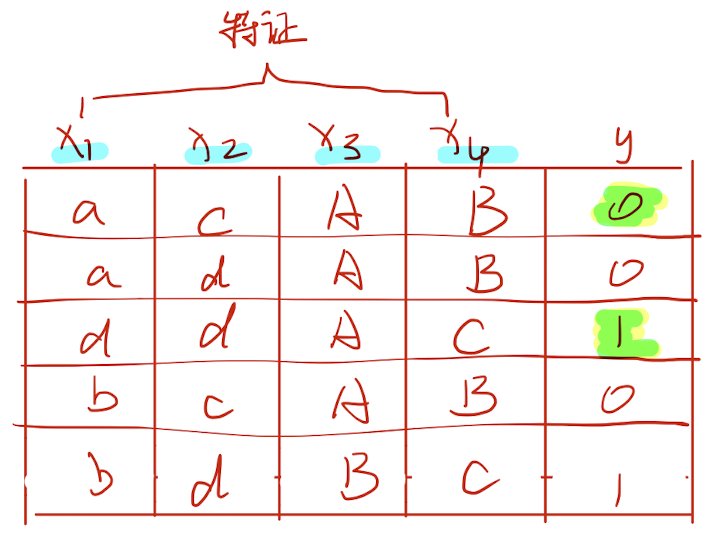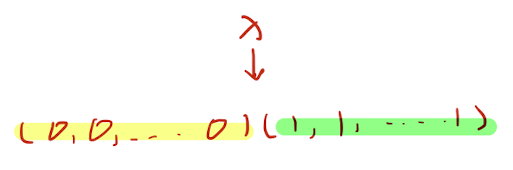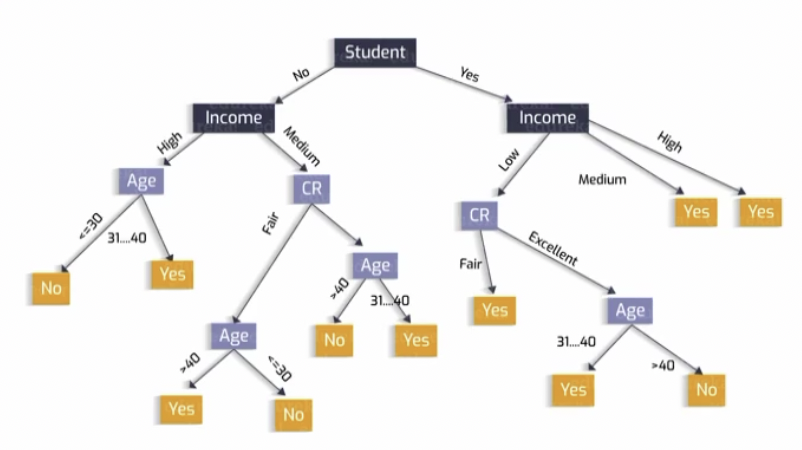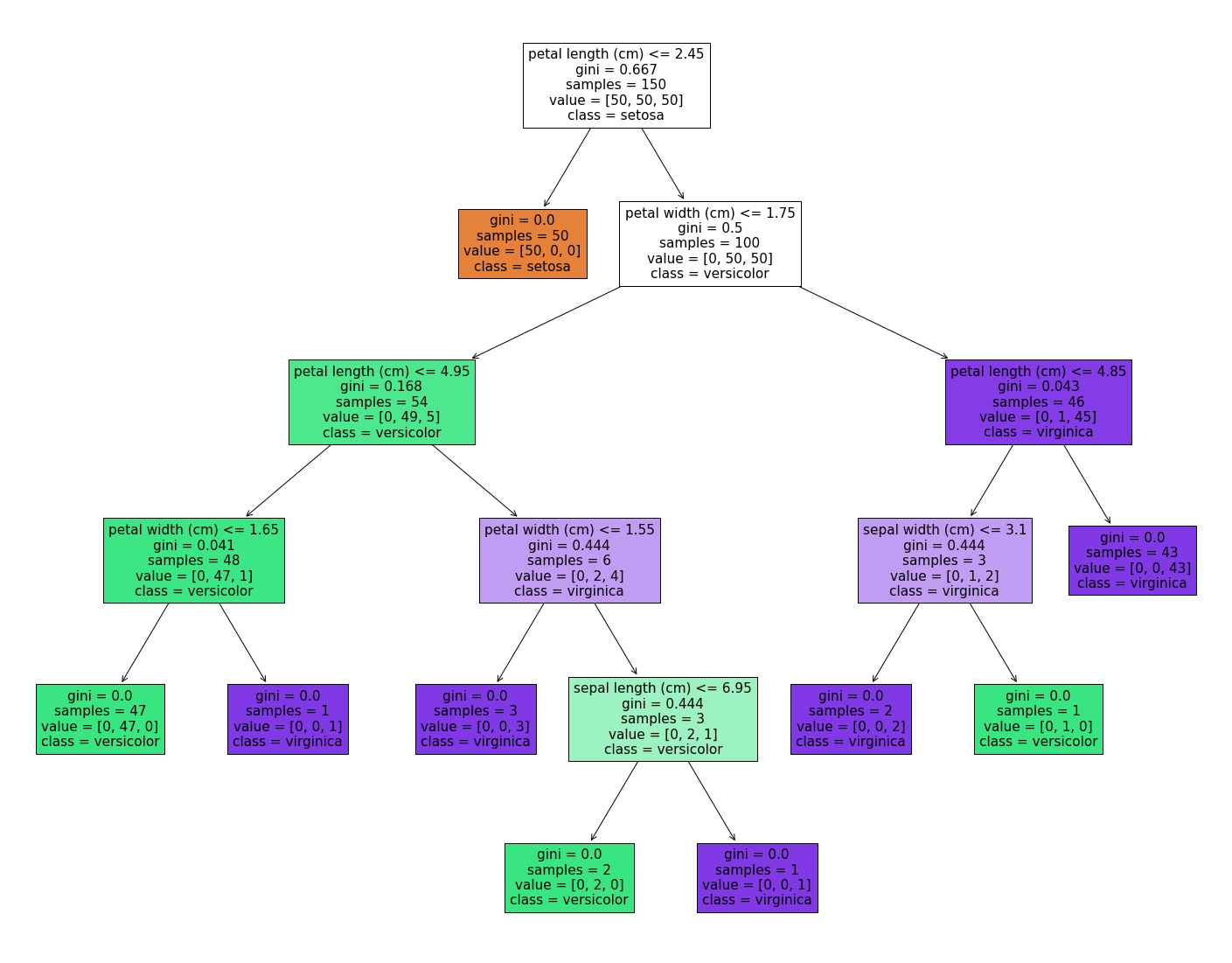# 16. 机器学习 - 决策树Hi，你好。我是茶桁。

## 决策树## 信息熵

\begin{align*} Entropy = \sum_{i=1}^n-p(c_i)log_2(p(c_i)) \end{align*}

## Gini 系数

\begin{align*} Gini = 1 - \sum_{i=1}^np^2(C_i) \\ \end{align*}

\begin{align*} loss = \frac{m_{left}}{n} \cdot G_{left} + \frac{m_{right}}{m} \cdot G_{right} \end{align*}

loss 函数的值最小的时候，就可以实现左右两边分的很均匀。我们把这个算法就叫做 CART。CART 算法其实就是classification and regression tree Algorithm, 也称为「分类和回归树算法」。

$J(k, t_k) = \frac{m_{left}}{m} \cdot MSE_{left} + \frac{m_{right}}{m}MSE_{right}$

MSE 是什么呢？其实就是均方误差。

\begin{align*} MSE_{node} & = \sum_{i \in node}(\hat y_{node} - y^{(i)})^2 \\ \hat y_{node} & = \frac{1}{m_{node}}\sum_{i\in node}y^{(i)} \end{align*}Hivan Du

2023-11-02

2023-11-25